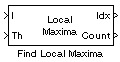Documentation

# Find Local Maxima

Find local maxima in matrices

• Library:
• Computer Vision Toolbox / Statistics## Description

The Find Local Maxima block finds the local maxima in a given input matrix. The block finds the local maxima of values within a region specified by the neighborhood size. The block compares the values in the search region to a user-specified threshold and identifies a value that is greater than or equal to the threshold as the local maximum. The threshold and the neighbourhood size must be chosen such that the block detects only the true local maxima and eliminates false peaks.

The input to this block can also be a Hough matrix generated from the Hough Transform block. The Find Local Maxima block outputs the one-based [x y] coordinates of the detected local maxima. The data sent to all input ports must be the same data type.

## Ports

### Input

expand all

Input, specified as the M-by-N matrix in which you want to detect the local maxima. If the input is a Hough matrix output from the Hough Transform block, select the Input is Hough matrix spanning full theta range parameter.

Data Types: `single` | `double` | `int8` | `int16` | `int32` | `uint8` | `uint16` | `uint32` | `fixed point`

Threshold for the detecting local maxima, specified as a scalar.

#### Dependencies

To enable this port, set the Source of threshold value parameter to `Input port`.

Data Types: `single` | `double` | `int8` | `int16` | `int32` | `uint8` | `uint16` | `uint32` | `fixed point`

### Output

expand all

Coordinates of the local maxima, returned as a P-by-2 matrix of one-based [x y] coordinates. P represents the number of local maxima.

Data Types: `single` | `double` | `uint8` | `uint16` | `uint32`

Number of local maxima, returned as a scalar.

#### Dependencies

To enable this port, clear the check box for the Output variable size signal parameter.

Data Types: `single` | `double` | `uint8` | `uint16` | `uint32`

## Parameters

expand all

Specify the maximum number of local maxima to detect in the input matrix. The value must be a positive integer greater than 0.

Specify the size of the neighborhood region as a two-element vector in the form ```[r c]```. The vector specifies the window size r×c, within which the block determines the local maxima. The window size must be greater than or equal to 1×1.

Specify the source for entering the threshold value. If you select `Input port`, the Th port is exposed on the block. If you select `Specify via dialog`, the Threshold parameter is exposed.

Specify a scalar value that represents the threshold for detecting the local maxima.

#### Dependencies

To enable this parameter, set the Source of threshold value parameter to `Specify via dialog`.

Select the parameter, if the input to this block is the Hough matrix output from the Hough Transform block. The Find Local Maxima block assumes that the input is a Hough matrix that is antisymmetric about the rho axis, with values of theta from -pi/2 to pi/2 radians. As a result, if the local maximum is near the boundary and its neighborhood region outside the Hough matrix, the block ignores the corresponding antisymmetric maximum.

Specify the data type for the coordinates of local maximums returned at the output port Idx.

Specify if the model supports input signals of varying length.

Specify the data type for the number of local maximums returned at the output port Count.

#### Dependencies

To enable this parameter, clear the Output variable size signal parameter.

## Block Characteristics

 Data Types `double` | `fixed point` | `integer` | `single` Multidimensional Signals `no` Variable-Size Signals `yes`